# Module: filters¶

 Apply hysteresis thresholding to image. skimage.filters.butterworth(image[, …]) Apply a Butterworth filter to enhance high or low frequency features. skimage.filters.correlate_sparse(image, kernel) Compute valid cross-correlation of padded_array and kernel. Find features between low_sigma and high_sigma in size. skimage.filters.farid(image, *[, mask]) Find the edge magnitude using the Farid transform. skimage.filters.farid_h(image, *[, mask]) Find the horizontal edges of an image using the Farid transform. skimage.filters.farid_v(image, *[, mask]) Find the vertical edges of an image using the Farid transform. skimage.filters.frangi(image[, sigmas, …]) Filter an image with the Frangi vesselness filter. skimage.filters.gabor(image, frequency[, …]) Return real and imaginary responses to Gabor filter. skimage.filters.gabor_kernel(frequency[, …]) Return complex 2D Gabor filter kernel. skimage.filters.gaussian(image[, sigma, …]) Multi-dimensional Gaussian filter. skimage.filters.hessian(image[, sigmas, …]) Filter an image with the Hybrid Hessian filter. skimage.filters.inverse(data[, …]) Apply the filter in reverse to the given data. skimage.filters.laplace(image[, ksize, mask]) Find the edges of an image using the Laplace operator. skimage.filters.median(image[, footprint, …]) Return local median of an image. skimage.filters.meijering(image[, sigmas, …]) Filter an image with the Meijering neuriteness filter. skimage.filters.prewitt(image[, mask, axis, …]) Find the edge magnitude using the Prewitt transform. skimage.filters.prewitt_h(image[, mask]) Find the horizontal edges of an image using the Prewitt transform. skimage.filters.prewitt_v(image[, mask]) Find the vertical edges of an image using the Prewitt transform. Return an image of the same shape where each pixel is the index of the pixel value in the ascending order of the unique values of image, aka the rank-order value. skimage.filters.roberts(image[, mask]) Find the edge magnitude using Roberts’ cross operator. skimage.filters.roberts_neg_diag(image[, mask]) Find the cross edges of an image using the Roberts’ Cross operator. skimage.filters.roberts_pos_diag(image[, mask]) Find the cross edges of an image using Roberts’ cross operator. skimage.filters.sato(image[, sigmas, …]) Filter an image with the Sato tubeness filter. skimage.filters.scharr(image[, mask, axis, …]) Find the edge magnitude using the Scharr transform. skimage.filters.scharr_h(image[, mask]) Find the horizontal edges of an image using the Scharr transform. skimage.filters.scharr_v(image[, mask]) Find the vertical edges of an image using the Scharr transform. skimage.filters.sobel(image[, mask, axis, …]) Find edges in an image using the Sobel filter. skimage.filters.sobel_h(image[, mask]) Find the horizontal edges of an image using the Sobel transform. skimage.filters.sobel_v(image[, mask]) Find the vertical edges of an image using the Sobel transform. skimage.filters.threshold_isodata([image, …]) Return threshold value(s) based on ISODATA method. skimage.filters.threshold_li(image, *[, …]) Compute threshold value by Li’s iterative Minimum Cross Entropy method. skimage.filters.threshold_local(image[, …]) Compute a threshold mask image based on local pixel neighborhood. Return threshold value based on the mean of grayscale values. skimage.filters.threshold_minimum([image, …]) Return threshold value based on minimum method. skimage.filters.threshold_multiotsu([image, …]) Generate classes-1 threshold values to divide gray levels in image, following Otsu’s method for multiple classes. skimage.filters.threshold_niblack(image[, …]) Applies Niblack local threshold to an array. skimage.filters.threshold_otsu([image, …]) Return threshold value based on Otsu’s method. skimage.filters.threshold_sauvola(image[, …]) Applies Sauvola local threshold to an array. skimage.filters.threshold_triangle(image[, …]) Return threshold value based on the triangle algorithm. skimage.filters.threshold_yen([image, …]) Return threshold value based on Yen’s method. skimage.filters.try_all_threshold(image[, …]) Returns a figure comparing the outputs of different thresholding methods. skimage.filters.unsharp_mask(image[, …]) Unsharp masking filter. skimage.filters.wiener(data[, …]) Minimum Mean Square Error (Wiener) inverse filter. skimage.filters.window(window_type, shape[, …]) Return an n-dimensional window of a given size and dimensionality. Linear Position-Invariant Filter (2-dimensional) skimage.filters.rank

## apply_hysteresis_threshold¶

skimage.filters.apply_hysteresis_threshold(image, low, high)[source]

Apply hysteresis thresholding to image.

This algorithm finds regions where image is greater than high OR image is greater than low and that region is connected to a region greater than high.

Parameters
imagearray, shape (M,[ N, …, P])

Grayscale input image.

lowfloat, or array of same shape as image

Lower threshold.

highfloat, or array of same shape as image

Higher threshold.

Returns
thresholdedarray of bool, same shape as image

Array in which True indicates the locations where image was above the hysteresis threshold.

References

1

J. Canny. A computational approach to edge detection. IEEE Transactions on Pattern Analysis and Machine Intelligence. 1986; vol. 8, pp.679-698. DOI:10.1109/TPAMI.1986.4767851

Examples

>>> image = np.array([1, 2, 3, 2, 1, 2, 1, 3, 2])
>>> apply_hysteresis_threshold(image, 1.5, 2.5).astype(int)
array([0, 1, 1, 1, 0, 0, 0, 1, 1])


### Examples using skimage.filters.apply_hysteresis_threshold¶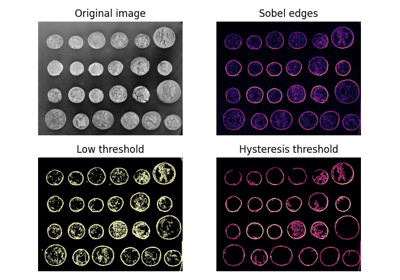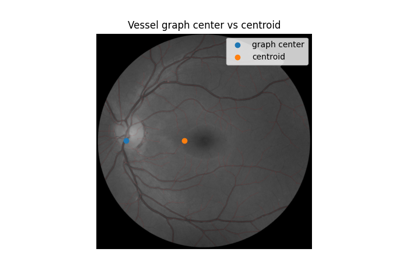## butterworth¶

skimage.filters.butterworth(image, cutoff_frequency_ratio=0.005, high_pass=True, order=2.0, channel_axis=None)[source]

Apply a Butterworth filter to enhance high or low frequency features.

This filter is defined in the Fourier domain.

Parameters
image(M[, N[, …, P]][, C]) ndarray

Input image.

cutoff_frequency_ratiofloat, optional

Determines the position of the cut-off relative to the shape of the FFT.

high_passbool, optional

Whether to perform a high pass filter. If False, a low pass filter is performed.

orderfloat, optional

Order of the filter which affects the slope near the cut-off. Higher order means steeper slope in frequency space.

channel_axisint, optional

If there is a channel dimension, provide the index here. If None (default) then all axes are assumed to be spatial dimensions.

Returns
resultndarray

The Butterworth-filtered image.

Notes

A band-pass filter can be achieved by combining a high pass and low pass filter.

The literature contains multiple conventions for the functional form of the Butterworth filter. Here it is implemented as the n-dimensional form of

$\frac{1}{1 - \left(\frac{f}{c*f_{max}}\right)^{2*n}}$

with $$f$$ the absolute value of the spatial frequency, $$c$$ the cutoff_frequency_ratio and $$n$$ the order modeled after 

References

1

Butterworth, Stephen. “On the theory of filter amplifiers.” Wireless Engineer 7.6 (1930): 536-541.

2

Russ, John C., et al. “The image processing handbook.” Computers in Physics 8.2 (1994): 177-178.

Examples

Apply a high pass and low pass Butterworth filter to a grayscale and color image respectively:

>>> from skimage.data import camera, astronaut
>>> from skimage.filters import butterworth
>>> high_pass = butterworth(camera(), 0.07, True, 8)
>>> low_pass = butterworth(astronaut(), 0.01, False, 4, channel_axis=-1)


## correlate_sparse¶

skimage.filters.correlate_sparse(image, kernel, mode='reflect')[source]

Compute valid cross-correlation of padded_array and kernel.

This function is fast when kernel is large with many zeros.

See scipy.ndimage.correlate for a description of cross-correlation.

Parameters
imagendarray, dtype float, shape (M, N,[ …,] P)

The input array. If mode is ‘valid’, this array should already be padded, as a margin of the same shape as kernel will be stripped off.

kernelndarray, dtype float shape (Q, R,[ …,] S)

The kernel to be correlated. Must have the same number of dimensions as padded_array. For high performance, it should be sparse (few nonzero entries).

modestring, optional

See scipy.ndimage.correlate for valid modes. Additionally, mode ‘valid’ is accepted, in which case no padding is applied and the result is the result for the smaller image for which the kernel is entirely inside the original data.

Returns
resultarray of float, shape (M, N,[ …,] P)

The result of cross-correlating image with kernel. If mode ‘valid’ is used, the resulting shape is (M-Q+1, N-R+1,[ …,] P-S+1).

## difference_of_gaussians¶

skimage.filters.difference_of_gaussians(image, low_sigma, high_sigma=None, *, mode='nearest', cval=0, channel_axis=None, truncate=4.0, multichannel=False)[source]

Find features between low_sigma and high_sigma in size.

This function uses the Difference of Gaussians method for applying band-pass filters to multi-dimensional arrays. The input array is blurred with two Gaussian kernels of differing sigmas to produce two intermediate, filtered images. The more-blurred image is then subtracted from the less-blurred image. The final output image will therefore have had high-frequency components attenuated by the smaller-sigma Gaussian, and low frequency components will have been removed due to their presence in the more-blurred intermediate.

Parameters
imagendarray

Input array to filter.

low_sigmascalar or sequence of scalars

Standard deviation(s) for the Gaussian kernel with the smaller sigmas across all axes. The standard deviations are given for each axis as a sequence, or as a single number, in which case the single number is used as the standard deviation value for all axes.

high_sigmascalar or sequence of scalars, optional (default is None)

Standard deviation(s) for the Gaussian kernel with the larger sigmas across all axes. The standard deviations are given for each axis as a sequence, or as a single number, in which case the single number is used as the standard deviation value for all axes. If None is given (default), sigmas for all axes are calculated as 1.6 * low_sigma.

mode{‘reflect’, ‘constant’, ‘nearest’, ‘mirror’, ‘wrap’}, optional

The mode parameter determines how the array borders are handled, where cval is the value when mode is equal to ‘constant’. Default is ‘nearest’.

cvalscalar, optional

Value to fill past edges of input if mode is ‘constant’. Default is 0.0

channel_axisint or None, optional

If None, the image is assumed to be a grayscale (single channel) image. Otherwise, this parameter indicates which axis of the array corresponds to channels.

New in version 0.19: channel_axis was added in 0.19.

truncatefloat, optional (default is 4.0)

Truncate the filter at this many standard deviations.

multichannelbool, optional (default: False)

Whether the last axis of the image is to be interpreted as multiple channels. If True, each channel is filtered separately (channels are not mixed together). This argument is deprecated: specify channel_axis instead.

Returns
filtered_imagendarray

the filtered array.

Other Parameters
multichannelDEPRECATED

Deprecated in favor of channel_axis.

Deprecated since version 0.19.

Notes

This function will subtract an array filtered with a Gaussian kernel with sigmas given by high_sigma from an array filtered with a Gaussian kernel with sigmas provided by low_sigma. The values for high_sigma must always be greater than or equal to the corresponding values in low_sigma, or a ValueError will be raised.

When high_sigma is none, the values for high_sigma will be calculated as 1.6x the corresponding values in low_sigma. This ratio was originally proposed by Marr and Hildreth (1980)  and is commonly used when approximating the inverted Laplacian of Gaussian, which is used in edge and blob detection.

Input image is converted according to the conventions of img_as_float.

Except for sigma values, all parameters are used for both filters.

References

1

Marr, D. and Hildreth, E. Theory of Edge Detection. Proc. R. Soc. Lond. Series B 207, 187-217 (1980). https://doi.org/10.1098/rspb.1980.0020

Examples

Apply a simple Difference of Gaussians filter to a color image:

>>> from skimage.data import astronaut
>>> from skimage.filters import difference_of_gaussians
>>> filtered_image = difference_of_gaussians(astronaut(), 2, 10,
...                                          channel_axis=-1)


Apply a Laplacian of Gaussian filter as approximated by the Difference of Gaussians filter:

>>> filtered_image = difference_of_gaussians(astronaut(), 2,
...                                          channel_axis=-1)


Apply a Difference of Gaussians filter to a grayscale image using different sigma values for each axis:

>>> from skimage.data import camera
>>> filtered_image = difference_of_gaussians(camera(), (2,5), (3,20))


### Examples using skimage.filters.difference_of_gaussians¶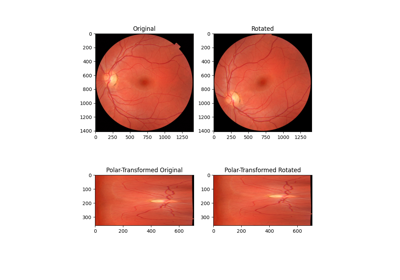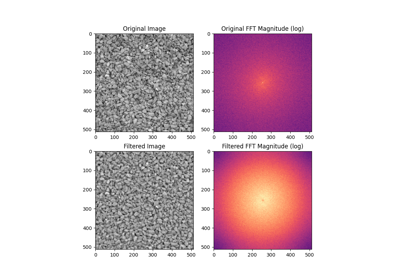## farid¶

skimage.filters.farid(image, *, mask=None)[source]

Find the edge magnitude using the Farid transform.

Parameters
image2-D array

Image to process.

An optional mask to limit the application to a certain area. Note that pixels surrounding masked regions are also masked to prevent masked regions from affecting the result.

Returns
output2-D array

The Farid edge map.

Notes

Take the square root of the sum of the squares of the horizontal and vertical derivatives to get a magnitude that is somewhat insensitive to direction. Similar to the Scharr operator, this operator is designed with a rotation invariance constraint.

References

1

Farid, H. and Simoncelli, E. P., “Differentiation of discrete multidimensional signals”, IEEE Transactions on Image Processing 13(4): 496-508, 2004. DOI:10.1109/TIP.2004.823819

2

Wikipedia, “Farid and Simoncelli Derivatives.” Available at: <https://en.wikipedia.org/wiki/Image_derivatives#Farid_and_Simoncelli_Derivatives>

Examples

>>> from skimage import data
>>> camera = data.camera()
>>> from skimage import filters
>>> edges = filters.farid(camera)


## farid_h¶

skimage.filters.farid_h(image, *, mask=None)[source]

Find the horizontal edges of an image using the Farid transform.

Parameters
image2-D array

Image to process.

An optional mask to limit the application to a certain area. Note that pixels surrounding masked regions are also masked to prevent masked regions from affecting the result.

Returns
output2-D array

The Farid edge map.

Notes

The kernel was constructed using the 5-tap weights from .

References

1

Farid, H. and Simoncelli, E. P., “Differentiation of discrete multidimensional signals”, IEEE Transactions on Image Processing 13(4): 496-508, 2004. DOI:10.1109/TIP.2004.823819

2

Farid, H. and Simoncelli, E. P. “Optimally rotation-equivariant directional derivative kernels”, In: 7th International Conference on Computer Analysis of Images and Patterns, Kiel, Germany. Sep, 1997.

### Examples using skimage.filters.farid_h¶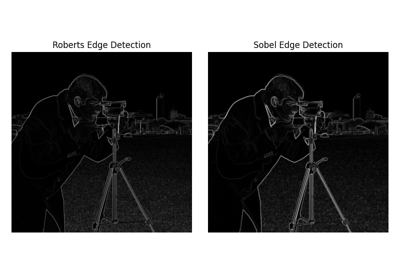## farid_v¶

skimage.filters.farid_v(image, *, mask=None)[source]

Find the vertical edges of an image using the Farid transform.

Parameters
image2-D array

Image to process.

An optional mask to limit the application to a certain area. Note that pixels surrounding masked regions are also masked to prevent masked regions from affecting the result.

Returns
output2-D array

The Farid edge map.

Notes

The kernel was constructed using the 5-tap weights from .

References

1

Farid, H. and Simoncelli, E. P., “Differentiation of discrete multidimensional signals”, IEEE Transactions on Image Processing 13(4): 496-508, 2004. DOI:10.1109/TIP.2004.823819

### Examples using skimage.filters.farid_v¶## frangi¶

skimage.filters.frangi(image, sigmas=range(1, 10, 2), scale_range=None, scale_step=None, alpha=0.5, beta=0.5, gamma=15, black_ridges=True, mode='reflect', cval=0)[source]

Filter an image with the Frangi vesselness filter.

This filter can be used to detect continuous ridges, e.g. vessels, wrinkles, rivers. It can be used to calculate the fraction of the whole image containing such objects.

Defined only for 2-D and 3-D images. Calculates the eigenvectors of the Hessian to compute the similarity of an image region to vessels, according to the method described in .

Parameters
image(N, M[, P]) ndarray

Array with input image data.

sigmasiterable of floats, optional

Sigmas used as scales of filter, i.e., np.arange(scale_range, scale_range, scale_step)

scale_range2-tuple of floats, optional

The range of sigmas used.

scale_stepfloat, optional

Step size between sigmas.

alphafloat, optional

Frangi correction constant that adjusts the filter’s sensitivity to deviation from a plate-like structure.

betafloat, optional

Frangi correction constant that adjusts the filter’s sensitivity to deviation from a blob-like structure.

gammafloat, optional

Frangi correction constant that adjusts the filter’s sensitivity to areas of high variance/texture/structure.

black_ridgesboolean, optional

When True (the default), the filter detects black ridges; when False, it detects white ridges.

mode{‘constant’, ‘reflect’, ‘wrap’, ‘nearest’, ‘mirror’}, optional

How to handle values outside the image borders.

cvalfloat, optional

Used in conjunction with mode ‘constant’, the value outside the image boundaries.

Returns
out(N, M[, P]) ndarray

Filtered image (maximum of pixels across all scales).

Notes

Written by Marc Schrijver, November 2001 Re-Written by D. J. Kroon, University of Twente, May 2009,  Adoption of 3D version from D. G. Ellis, Januar 20017, 

References

1

Frangi, A. F., Niessen, W. J., Vincken, K. L., & Viergever, M. A. (1998,). Multiscale vessel enhancement filtering. In International Conference on Medical Image Computing and Computer-Assisted Intervention (pp. 130-137). Springer Berlin Heidelberg. DOI:10.1007/BFb0056195

2

Kroon, D. J.: Hessian based Frangi vesselness filter.

3

### Examples using skimage.filters.frangi¶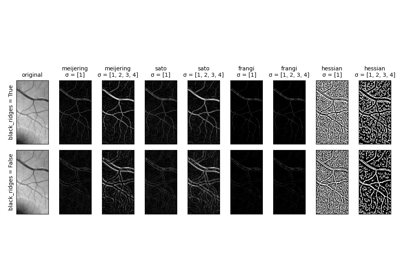## gabor¶

skimage.filters.gabor(image, frequency, theta=0, bandwidth=1, sigma_x=None, sigma_y=None, n_stds=3, offset=0, mode='reflect', cval=0)[source]

Return real and imaginary responses to Gabor filter.

The real and imaginary parts of the Gabor filter kernel are applied to the image and the response is returned as a pair of arrays.

Gabor filter is a linear filter with a Gaussian kernel which is modulated by a sinusoidal plane wave. Frequency and orientation representations of the Gabor filter are similar to those of the human visual system. Gabor filter banks are commonly used in computer vision and image processing. They are especially suitable for edge detection and texture classification.

Parameters
image2-D array

Input image.

frequencyfloat

Spatial frequency of the harmonic function. Specified in pixels.

thetafloat, optional

Orientation in radians. If 0, the harmonic is in the x-direction.

bandwidthfloat, optional

The bandwidth captured by the filter. For fixed bandwidth, sigma_x and sigma_y will decrease with increasing frequency. This value is ignored if sigma_x and sigma_y are set by the user.

sigma_x, sigma_yfloat, optional

Standard deviation in x- and y-directions. These directions apply to the kernel before rotation. If theta = pi/2, then the kernel is rotated 90 degrees so that sigma_x controls the vertical direction.

n_stdsscalar, optional

The linear size of the kernel is n_stds (3 by default) standard deviations.

offsetfloat, optional

Phase offset of harmonic function in radians.

mode{‘constant’, ‘nearest’, ‘reflect’, ‘mirror’, ‘wrap’}, optional

Mode used to convolve image with a kernel, passed to ndi.convolve

cvalscalar, optional

Value to fill past edges of input if mode of convolution is ‘constant’. The parameter is passed to ndi.convolve.

Returns
real, imagarrays

Filtered images using the real and imaginary parts of the Gabor filter kernel. Images are of the same dimensions as the input one.

References

1

https://en.wikipedia.org/wiki/Gabor_filter

2

https://web.archive.org/web/20180127125930/http://mplab.ucsd.edu/tutorials/gabor.pdf

Examples

>>> from skimage.filters import gabor
>>> from skimage import data, io
>>> from matplotlib import pyplot as plt

>>> image = data.coins()
>>> # detecting edges in a coin image
>>> filt_real, filt_imag = gabor(image, frequency=0.6)
>>> plt.figure()
>>> io.imshow(filt_real)
>>> io.show()

>>> # less sensitivity to finer details with the lower frequency kernel
>>> filt_real, filt_imag = gabor(image, frequency=0.1)
>>> plt.figure()
>>> io.imshow(filt_real)
>>> io.show()


## gabor_kernel¶

skimage.filters.gabor_kernel(frequency, theta=0, bandwidth=1, sigma_x=None, sigma_y=None, n_stds=3, offset=0, dtype=<class 'numpy.complex128'>)[source]

Return complex 2D Gabor filter kernel.

Gabor kernel is a Gaussian kernel modulated by a complex harmonic function. Harmonic function consists of an imaginary sine function and a real cosine function. Spatial frequency is inversely proportional to the wavelength of the harmonic and to the standard deviation of a Gaussian kernel. The bandwidth is also inversely proportional to the standard deviation.

Parameters
frequencyfloat

Spatial frequency of the harmonic function. Specified in pixels.

thetafloat, optional

Orientation in radians. If 0, the harmonic is in the x-direction.

bandwidthfloat, optional

The bandwidth captured by the filter. For fixed bandwidth, sigma_x and sigma_y will decrease with increasing frequency. This value is ignored if sigma_x and sigma_y are set by the user.

sigma_x, sigma_yfloat, optional

Standard deviation in x- and y-directions. These directions apply to the kernel before rotation. If theta = pi/2, then the kernel is rotated 90 degrees so that sigma_x controls the vertical direction.

n_stdsscalar, optional

The linear size of the kernel is n_stds (3 by default) standard deviations

offsetfloat, optional

Phase offset of harmonic function in radians.

dtype{np.complex64, np.complex128}

Specifies if the filter is single or double precision complex.

Returns
gcomplex array

Complex filter kernel.

References

1

https://en.wikipedia.org/wiki/Gabor_filter

2

https://web.archive.org/web/20180127125930/http://mplab.ucsd.edu/tutorials/gabor.pdf

Examples

>>> from skimage.filters import gabor_kernel
>>> from skimage import io
>>> from matplotlib import pyplot as plt

>>> gk = gabor_kernel(frequency=0.2)
>>> plt.figure()
>>> io.imshow(gk.real)
>>> io.show()

>>> # more ripples (equivalent to increasing the size of the
>>> gk = gabor_kernel(frequency=0.2, bandwidth=0.1)
>>> plt.figure()
>>> io.imshow(gk.real)
>>> io.show()


### Examples using skimage.filters.gabor_kernel¶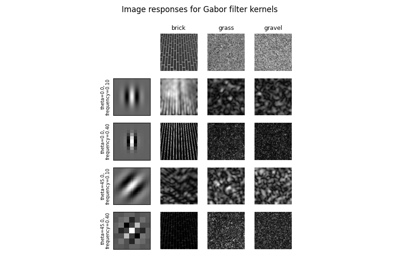## gaussian¶

skimage.filters.gaussian(image, sigma=1, output=None, mode='nearest', cval=0, multichannel=None, preserve_range=False, truncate=4.0, *, channel_axis=None)[source]

Multi-dimensional Gaussian filter.

Parameters
imagearray-like

Input image (grayscale or color) to filter.

sigmascalar or sequence of scalars, optional

Standard deviation for Gaussian kernel. The standard deviations of the Gaussian filter are given for each axis as a sequence, or as a single number, in which case it is equal for all axes.

outputarray, optional

The output parameter passes an array in which to store the filter output.

mode{‘reflect’, ‘constant’, ‘nearest’, ‘mirror’, ‘wrap’}, optional

The mode parameter determines how the array borders are handled, where cval is the value when mode is equal to ‘constant’. Default is ‘nearest’.

cvalscalar, optional

Value to fill past edges of input if mode is ‘constant’. Default is 0.0

multichannelbool, optional (default: None)

Whether the last axis of the image is to be interpreted as multiple channels. If True, each channel is filtered separately (channels are not mixed together). Only 3 channels are supported. If None, the function will attempt to guess this, and raise a warning if ambiguous, when the array has shape (M, N, 3). This argument is deprecated: specify channel_axis instead.

preserve_rangebool, optional

If True, keep the original range of values. Otherwise, the input image is converted according to the conventions of img_as_float (Normalized first to values [-1.0 ; 1.0] or [0 ; 1.0] depending on dtype of input)

truncatefloat, optional

Truncate the filter at this many standard deviations.

channel_axisint or None, optional

If None, the image is assumed to be a grayscale (single channel) image. Otherwise, this parameter indicates which axis of the array corresponds to channels.

New in version 0.19: channel_axis was added in 0.19.

Returns
filtered_imagendarray

the filtered array

Other Parameters
multichannelDEPRECATED

Deprecated in favor of channel_axis.

Deprecated since version 0.19.

Notes

This function is a wrapper around scipy.ndi.gaussian_filter().

Integer arrays are converted to float.

The output should be floating point data type since gaussian converts to float provided image. If output is not provided, another array will be allocated and returned as the result.

The multi-dimensional filter is implemented as a sequence of one-dimensional convolution filters. The intermediate arrays are stored in the same data type as the output. Therefore, for output types with a limited precision, the results may be imprecise because intermediate results may be stored with insufficient precision.

Examples

>>> a = np.zeros((3, 3))
>>> a[1, 1] = 1
>>> a
array([[0., 0., 0.],
[0., 1., 0.],
[0., 0., 0.]])
>>> gaussian(a, sigma=0.4)  # mild smoothing
array([[0.00163116, 0.03712502, 0.00163116],
[0.03712502, 0.84496158, 0.03712502],
[0.00163116, 0.03712502, 0.00163116]])
>>> gaussian(a, sigma=1)  # more smoothing
array([[0.05855018, 0.09653293, 0.05855018],
[0.09653293, 0.15915589, 0.09653293],
[0.05855018, 0.09653293, 0.05855018]])
>>> # Several modes are possible for handling boundaries
>>> gaussian(a, sigma=1, mode='reflect')
array([[0.08767308, 0.12075024, 0.08767308],
[0.12075024, 0.16630671, 0.12075024],
[0.08767308, 0.12075024, 0.08767308]])
>>> # For RGB images, each is filtered separately
>>> from skimage.data import astronaut
>>> image = astronaut()
>>> filtered_img = gaussian(image, sigma=1, channel_axis=-1)


### Examples using skimage.filters.gaussian¶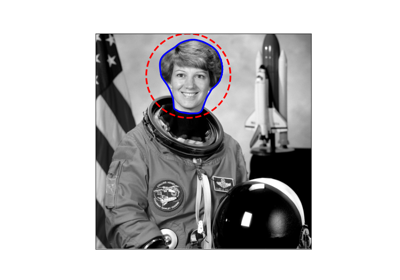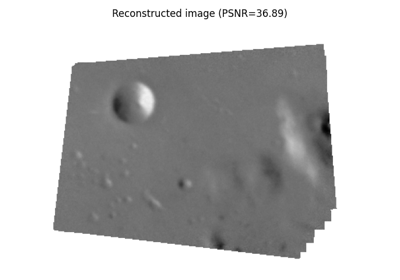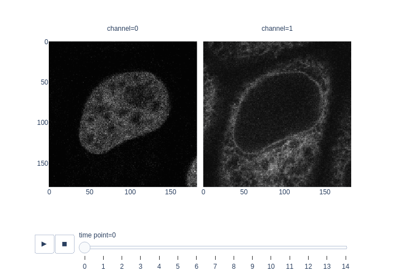## hessian¶

skimage.filters.hessian(image, sigmas=range(1, 10, 2), scale_range=None, scale_step=None, alpha=0.5, beta=0.5, gamma=15, black_ridges=True, mode='reflect', cval=0)[source]

Filter an image with the Hybrid Hessian filter.

This filter can be used to detect continuous edges, e.g. vessels, wrinkles, rivers. It can be used to calculate the fraction of the whole image containing such objects.

Defined only for 2-D and 3-D images. Almost equal to Frangi filter, but uses alternative method of smoothing. Refer to  to find the differences between Frangi and Hessian filters.

Parameters
image(N, M[, P]) ndarray

Array with input image data.

sigmasiterable of floats, optional

Sigmas used as scales of filter, i.e., np.arange(scale_range, scale_range, scale_step)

scale_range2-tuple of floats, optional

The range of sigmas used.

scale_stepfloat, optional

Step size between sigmas.

betafloat, optional

Frangi correction constant that adjusts the filter’s sensitivity to deviation from a blob-like structure.

gammafloat, optional

Frangi correction constant that adjusts the filter’s sensitivity to areas of high variance/texture/structure.

black_ridgesboolean, optional

When True (the default), the filter detects black ridges; when False, it detects white ridges.

mode{‘constant’, ‘reflect’, ‘wrap’, ‘nearest’, ‘mirror’}, optional

How to handle values outside the image borders.

cvalfloat, optional

Used in conjunction with mode ‘constant’, the value outside the image boundaries.

Returns
out(N, M[, P]) ndarray

Filtered image (maximum of pixels across all scales).

Notes

Written by Marc Schrijver (November 2001) Re-Written by D. J. Kroon University of Twente (May 2009) 

References

1

Ng, C. C., Yap, M. H., Costen, N., & Li, B. (2014,). Automatic wrinkle detection using hybrid Hessian filter. In Asian Conference on Computer Vision (pp. 609-622). Springer International Publishing. DOI:10.1007/978-3-319-16811-1_40

2

Kroon, D. J.: Hessian based Frangi vesselness filter.

### Examples using skimage.filters.hessian¶## inverse¶

skimage.filters.inverse(data, impulse_response=None, filter_params={}, max_gain=2, predefined_filter=None)[source]

Apply the filter in reverse to the given data.

Parameters
data(M,N) ndarray

Input data.

impulse_responsecallable f(r, c, **filter_params)

Impulse response of the filter. See LPIFilter2D.__init__.

filter_paramsdict

Additional keyword parameters to the impulse_response function.

max_gainfloat

Limit the filter gain. Often, the filter contains zeros, which would cause the inverse filter to have infinite gain. High gain causes amplification of artefacts, so a conservative limit is recommended.

Other Parameters
predefined_filterLPIFilter2D

If you need to apply the same filter multiple times over different images, construct the LPIFilter2D and specify it here.

## laplace¶

skimage.filters.laplace(image, ksize=3, mask=None)[source]

Find the edges of an image using the Laplace operator.

Parameters
imagendarray

Image to process.

ksizeint, optional

Define the size of the discrete Laplacian operator such that it will have a size of (ksize,) * image.ndim.

An optional mask to limit the application to a certain area. Note that pixels surrounding masked regions are also masked to prevent masked regions from affecting the result.

Returns
outputndarray

The Laplace edge map.

Notes

The Laplacian operator is generated using the function skimage.restoration.uft.laplacian().

## median¶

skimage.filters.median(image, footprint=None, out=None, mode='nearest', cval=0.0, behavior='ndimage')[source]

Return local median of an image.

Parameters
imagearray-like

Input image.

footprintndarray, optional

If behavior=='rank', footprint is a 2-D array of 1’s and 0’s. If behavior=='ndimage', footprint is a N-D array of 1’s and 0’s with the same number of dimension than image. If None, footprint will be a N-D array with 3 elements for each dimension (e.g., vector, square, cube, etc.)

outndarray, (same dtype as image), optional

If None, a new array is allocated.

mode{‘reflect’, ‘constant’, ‘nearest’, ‘mirror’,’‘wrap’}, optional

The mode parameter determines how the array borders are handled, where cval is the value when mode is equal to ‘constant’. Default is ‘nearest’.

New in version 0.15: mode is used when behavior='ndimage'.

cvalscalar, optional

Value to fill past edges of input if mode is ‘constant’. Default is 0.0

New in version 0.15: cval was added in 0.15 is used when behavior='ndimage'.

behavior{‘ndimage’, ‘rank’}, optional

Either to use the old behavior (i.e., < 0.15) or the new behavior. The old behavior will call the skimage.filters.rank.median(). The new behavior will call the scipy.ndimage.median_filter(). Default is ‘ndimage’.

New in version 0.15: behavior is introduced in 0.15

Changed in version 0.16: Default behavior has been changed from ‘rank’ to ‘ndimage’

Returns
out2-D array (same dtype as input image)

Output image.

Other Parameters
selemDEPRECATED

Deprecated in favor of footprint.

Deprecated since version 0.19.

skimage.filters.rank.median()

Rank-based implementation of the median filtering offering more flexibility with additional parameters but dedicated for unsigned integer images.

Examples

>>> from skimage import data
>>> from skimage.morphology import disk
>>> from skimage.filters import median
>>> img = data.camera()
>>> med = median(img, disk(5))


## meijering¶

skimage.filters.meijering(image, sigmas=range(1, 10, 2), alpha=None, black_ridges=True, mode='reflect', cval=0)[source]

Filter an image with the Meijering neuriteness filter.

This filter can be used to detect continuous ridges, e.g. neurites, wrinkles, rivers. It can be used to calculate the fraction of the whole image containing such objects.

Calculates the eigenvectors of the Hessian to compute the similarity of an image region to neurites, according to the method described in .

Parameters
image(N, M[, …, P]) ndarray

Array with input image data.

sigmasiterable of floats, optional

Sigmas used as scales of filter

alphafloat, optional

Frangi correction constant that adjusts the filter’s sensitivity to deviation from a plate-like structure.

black_ridgesboolean, optional

When True (the default), the filter detects black ridges; when False, it detects white ridges.

mode{‘constant’, ‘reflect’, ‘wrap’, ‘nearest’, ‘mirror’}, optional

How to handle values outside the image borders.

cvalfloat, optional

Used in conjunction with mode ‘constant’, the value outside the image boundaries.

Returns
out(N, M[, …, P]) ndarray

Filtered image (maximum of pixels across all scales).

References

1

Meijering, E., Jacob, M., Sarria, J. C., Steiner, P., Hirling, H., Unser, M. (2004). Design and validation of a tool for neurite tracing and analysis in fluorescence microscopy images. Cytometry Part A, 58(2), 167-176. DOI:10.1002/cyto.a.20022

### Examples using skimage.filters.meijering¶## prewitt¶

skimage.filters.prewitt(image, mask=None, *, axis=None, mode='reflect', cval=0.0)[source]

Find the edge magnitude using the Prewitt transform.

Parameters
imagearray

The input image.

Clip the output image to this mask. (Values where mask=0 will be set to 0.)

axisint or sequence of int, optional

Compute the edge filter along this axis. If not provided, the edge magnitude is computed. This is defined as:

prw_mag = np.sqrt(sum([prewitt(image, axis=i)**2
for i in range(image.ndim)]) / image.ndim)


The magnitude is also computed if axis is a sequence.

modestr or sequence of str, optional

The boundary mode for the convolution. See scipy.ndimage.convolve for a description of the modes. This can be either a single boundary mode or one boundary mode per axis.

cvalfloat, optional

When mode is 'constant', this is the constant used in values outside the boundary of the image data.

Returns
outputarray of float

The Prewitt edge map.

Notes

The edge magnitude depends slightly on edge directions, since the approximation of the gradient operator by the Prewitt operator is not completely rotation invariant. For a better rotation invariance, the Scharr operator should be used. The Sobel operator has a better rotation invariance than the Prewitt operator, but a worse rotation invariance than the Scharr operator.

Examples

>>> from skimage import data
>>> from skimage import filters
>>> camera = data.camera()
>>> edges = filters.prewitt(camera)


### Examples using skimage.filters.prewitt¶## prewitt_h¶

skimage.filters.prewitt_h(image, mask=None)[source]

Find the horizontal edges of an image using the Prewitt transform.

Parameters
image2-D array

Image to process.

An optional mask to limit the application to a certain area. Note that pixels surrounding masked regions are also masked to prevent masked regions from affecting the result.

Returns
output2-D array

The Prewitt edge map.

Notes

We use the following kernel:

 1/3   1/3   1/3
0     0     0
-1/3  -1/3  -1/3


### Examples using skimage.filters.prewitt_h¶## prewitt_v¶

skimage.filters.prewitt_v(image, mask=None)[source]

Find the vertical edges of an image using the Prewitt transform.

Parameters
image2-D array

Image to process.

An optional mask to limit the application to a certain area. Note that pixels surrounding masked regions are also masked to prevent masked regions from affecting the result.

Returns
output2-D array

The Prewitt edge map.

Notes

We use the following kernel:

1/3   0  -1/3
1/3   0  -1/3
1/3   0  -1/3


### Examples using skimage.filters.prewitt_v¶## rank_order¶

skimage.filters.rank_order(image)[source]

Return an image of the same shape where each pixel is the index of the pixel value in the ascending order of the unique values of image, aka the rank-order value.

Parameters
imagendarray
Returns
labelsndarray of type np.uint32, of shape image.shape

New array where each pixel has the rank-order value of the corresponding pixel in image. Pixel values are between 0 and n - 1, where n is the number of distinct unique values in image.

original_values1-D ndarray

Unique original values of image

Examples

>>> a = np.array([[1, 4, 5], [4, 4, 1], [5, 1, 1]])
>>> a
array([[1, 4, 5],
[4, 4, 1],
[5, 1, 1]])
>>> rank_order(a)
(array([[0, 1, 2],
[1, 1, 0],
[2, 0, 0]], dtype=uint32), array([1, 4, 5]))
>>> b = np.array([-1., 2.5, 3.1, 2.5])
>>> rank_order(b)
(array([0, 1, 2, 1], dtype=uint32), array([-1. ,  2.5,  3.1]))


## roberts¶

skimage.filters.roberts(image, mask=None)[source]

Find the edge magnitude using Roberts’ cross operator.

Parameters
image2-D array

Image to process.

An optional mask to limit the application to a certain area. Note that pixels surrounding masked regions are also masked to prevent masked regions from affecting the result.

Returns
output2-D array

The Roberts’ Cross edge map.

Examples

>>> from skimage import data
>>> camera = data.camera()
>>> from skimage import filters
>>> edges = filters.roberts(camera)


### Examples using skimage.filters.roberts¶## roberts_neg_diag¶

skimage.filters.roberts_neg_diag(image, mask=None)[source]

Find the cross edges of an image using the Roberts’ Cross operator.

The kernel is applied to the input image to produce separate measurements of the gradient component one orientation.

Parameters
image2-D array

Image to process.

An optional mask to limit the application to a certain area. Note that pixels surrounding masked regions are also masked to prevent masked regions from affecting the result.

Returns
output2-D array

The Robert’s edge map.

Notes

We use the following kernel:

 0   1
-1   0


## roberts_pos_diag¶

skimage.filters.roberts_pos_diag(image, mask=None)[source]

Find the cross edges of an image using Roberts’ cross operator.

The kernel is applied to the input image to produce separate measurements of the gradient component one orientation.

Parameters
image2-D array

Image to process.

An optional mask to limit the application to a certain area. Note that pixels surrounding masked regions are also masked to prevent masked regions from affecting the result.

Returns
output2-D array

The Robert’s edge map.

Notes

We use the following kernel:

1   0
0  -1


## sato¶

skimage.filters.sato(image, sigmas=range(1, 10, 2), black_ridges=True, mode='reflect', cval=0)[source]

Filter an image with the Sato tubeness filter.

This filter can be used to detect continuous ridges, e.g. tubes, wrinkles, rivers. It can be used to calculate the fraction of the whole image containing such objects.

Defined only for 2-D and 3-D images. Calculates the eigenvectors of the Hessian to compute the similarity of an image region to tubes, according to the method described in .

Parameters
image(N, M[, P]) ndarray

Array with input image data.

sigmasiterable of floats, optional

Sigmas used as scales of filter.

black_ridgesboolean, optional

When True (the default), the filter detects black ridges; when False, it detects white ridges.

mode{‘constant’, ‘reflect’, ‘wrap’, ‘nearest’, ‘mirror’}, optional

How to handle values outside the image borders.

cvalfloat, optional

Used in conjunction with mode ‘constant’, the value outside the image boundaries.

Returns
out(N, M[, P]) ndarray

Filtered image (maximum of pixels across all scales).

References

1

Sato, Y., Nakajima, S., Shiraga, N., Atsumi, H., Yoshida, S., Koller, T., …, Kikinis, R. (1998). Three-dimensional multi-scale line filter for segmentation and visualization of curvilinear structures in medical images. Medical image analysis, 2(2), 143-168. DOI:10.1016/S1361-8415(98)80009-1

### Examples using skimage.filters.sato¶## scharr¶

skimage.filters.scharr(image, mask=None, *, axis=None, mode='reflect', cval=0.0)[source]

Find the edge magnitude using the Scharr transform.

Parameters
imagearray

The input image.

Clip the output image to this mask. (Values where mask=0 will be set to 0.)

axisint or sequence of int, optional

Compute the edge filter along this axis. If not provided, the edge magnitude is computed. This is defined as:

sch_mag = np.sqrt(sum([scharr(image, axis=i)**2
for i in range(image.ndim)]) / image.ndim)


The magnitude is also computed if axis is a sequence.

modestr or sequence of str, optional

The boundary mode for the convolution. See scipy.ndimage.convolve for a description of the modes. This can be either a single boundary mode or one boundary mode per axis.

cvalfloat, optional

When mode is 'constant', this is the constant used in values outside the boundary of the image data.

Returns
outputarray of float

The Scharr edge map.

Notes

The Scharr operator has a better rotation invariance than other edge filters such as the Sobel or the Prewitt operators.

References

1

D. Kroon, 2009, Short Paper University Twente, Numerical Optimization of Kernel Based Image Derivatives.

2

https://en.wikipedia.org/wiki/Sobel_operator#Alternative_operators

Examples

>>> from skimage import data
>>> from skimage import filters
>>> camera = data.camera()
>>> edges = filters.scharr(camera)


### Examples using skimage.filters.scharr¶## scharr_h¶

skimage.filters.scharr_h(image, mask=None)[source]

Find the horizontal edges of an image using the Scharr transform.

Parameters
image2-D array

Image to process.

An optional mask to limit the application to a certain area. Note that pixels surrounding masked regions are also masked to prevent masked regions from affecting the result.

Returns
output2-D array

The Scharr edge map.

Notes

We use the following kernel:

 3   10   3
0    0   0
-3  -10  -3


References

1

D. Kroon, 2009, Short Paper University Twente, Numerical Optimization of Kernel Based Image Derivatives.

### Examples using skimage.filters.scharr_h¶## scharr_v¶

skimage.filters.scharr_v(image, mask=None)[source]

Find the vertical edges of an image using the Scharr transform.

Parameters
image2-D array

Image to process

An optional mask to limit the application to a certain area. Note that pixels surrounding masked regions are also masked to prevent masked regions from affecting the result.

Returns
output2-D array

The Scharr edge map.

Notes

We use the following kernel:

 3   0   -3
10   0  -10
3   0   -3


References

1

D. Kroon, 2009, Short Paper University Twente, Numerical Optimization of Kernel Based Image Derivatives.

### Examples using skimage.filters.scharr_v¶## sobel¶

skimage.filters.sobel(image, mask=None, *, axis=None, mode='reflect', cval=0.0)[source]

Find edges in an image using the Sobel filter.

Parameters
imagearray

The input image.

Clip the output image to this mask. (Values where mask=0 will be set to 0.)

axisint or sequence of int, optional

Compute the edge filter along this axis. If not provided, the edge magnitude is computed. This is defined as:

sobel_mag = np.sqrt(sum([sobel(image, axis=i)**2
for i in range(image.ndim)]) / image.ndim)


The magnitude is also computed if axis is a sequence.

modestr or sequence of str, optional

The boundary mode for the convolution. See scipy.ndimage.convolve for a description of the modes. This can be either a single boundary mode or one boundary mode per axis.

cvalfloat, optional

When mode is 'constant', this is the constant used in values outside the boundary of the image data.

Returns
outputarray of float

The Sobel edge map.

References

1

D. Kroon, 2009, Short Paper University Twente, Numerical Optimization of Kernel Based Image Derivatives.

2

https://en.wikipedia.org/wiki/Sobel_operator

Examples

>>> from skimage import data
>>> from skimage import filters
>>> camera = data.camera()
>>> edges = filters.sobel(camera)


### Examples using skimage.filters.sobel¶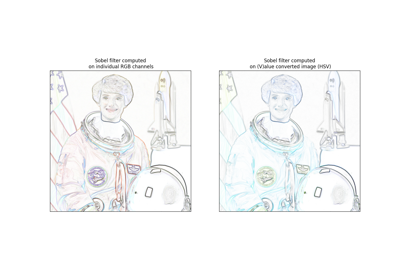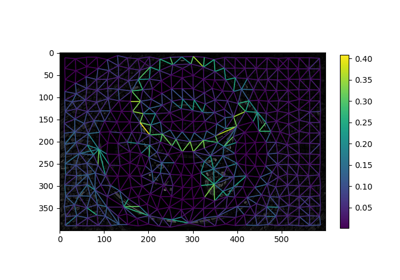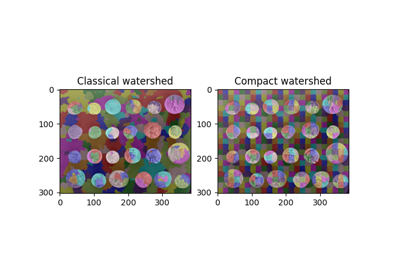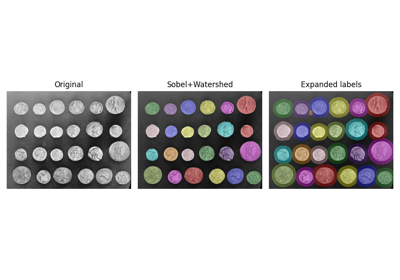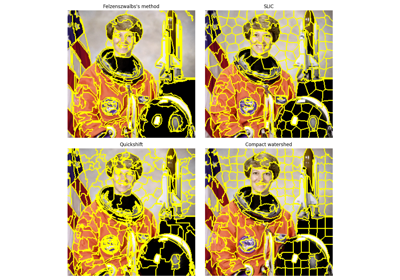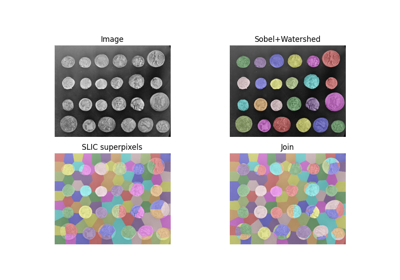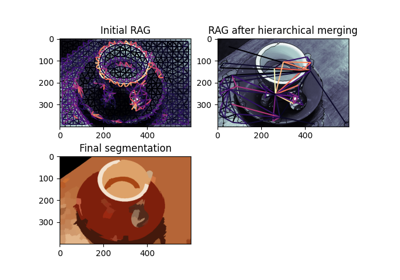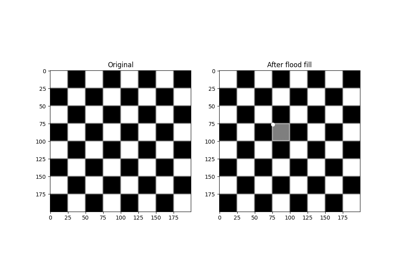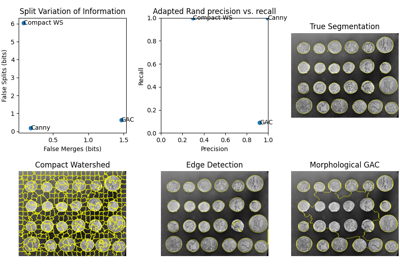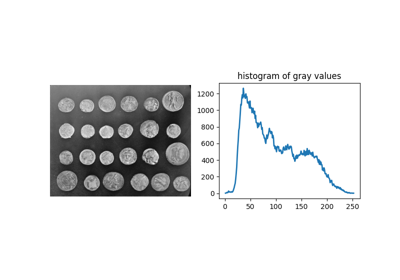## sobel_h¶

skimage.filters.sobel_h(image, mask=None)[source]

Find the horizontal edges of an image using the Sobel transform.

Parameters
image2-D array

Image to process.

An optional mask to limit the application to a certain area. Note that pixels surrounding masked regions are also masked to prevent masked regions from affecting the result.

Returns
output2-D array

The Sobel edge map.

Notes

We use the following kernel:

 1   2   1
0   0   0
-1  -2  -1


### Examples using skimage.filters.sobel_h¶## sobel_v¶

skimage.filters.sobel_v(image, mask=None)[source]

Find the vertical edges of an image using the Sobel transform.

Parameters
image2-D array

Image to process.

An optional mask to limit the application to a certain area. Note that pixels surrounding masked regions are also masked to prevent masked regions from affecting the result.

Returns
output2-D array

The Sobel edge map.

Notes

We use the following kernel:

1   0  -1
2   0  -2
1   0  -1


### Examples using skimage.filters.sobel_v¶## threshold_isodata¶

skimage.filters.threshold_isodata(image=None, nbins=256, return_all=False, *, hist=None)[source]

Return threshold value(s) based on ISODATA method.

Histogram-based threshold, known as Ridler-Calvard method or inter-means. Threshold values returned satisfy the following equality:

threshold = (image[image <= threshold].mean() +
image[image > threshold].mean()) / 2.0


That is, returned thresholds are intensities that separate the image into two groups of pixels, where the threshold intensity is midway between the mean intensities of these groups.

For integer images, the above equality holds to within one; for floating- point images, the equality holds to within the histogram bin-width.

Either image or hist must be provided. In case hist is given, the actual histogram of the image is ignored.

Parameters
image(N, M[, …, P]) ndarray

Grayscale input image.

nbinsint, optional

Number of bins used to calculate histogram. This value is ignored for integer arrays.

return_allbool, optional

If False (default), return only the lowest threshold that satisfies the above equality. If True, return all valid thresholds.

histarray, or 2-tuple of arrays, optional

Histogram to determine the threshold from and a corresponding array of bin center intensities. Alternatively, only the histogram can be passed.

Returns
thresholdfloat or int or array

Threshold value(s).

References

1

Ridler, TW & Calvard, S (1978), “Picture thresholding using an iterative selection method” IEEE Transactions on Systems, Man and Cybernetics 8: 630-632, DOI:10.1109/TSMC.1978.4310039

2

Sezgin M. and Sankur B. (2004) “Survey over Image Thresholding Techniques and Quantitative Performance Evaluation” Journal of Electronic Imaging, 13(1): 146-165, http://www.busim.ee.boun.edu.tr/~sankur/SankurFolder/Threshold_survey.pdf DOI:10.1117/1.1631315

3

ImageJ AutoThresholder code, http://fiji.sc/wiki/index.php/Auto_Threshold

Examples

>>> from skimage.data import coins
>>> image = coins()
>>> thresh = threshold_isodata(image)
>>> binary = image > thresh


## threshold_li¶

skimage.filters.threshold_li(image, *, tolerance=None, initial_guess=None, iter_callback=None)[source]

Compute threshold value by Li’s iterative Minimum Cross Entropy method.

Parameters
image(N, M[, …, P]) ndarray

Grayscale input image.

tolerancefloat, optional

Finish the computation when the change in the threshold in an iteration is less than this value. By default, this is half the smallest difference between intensity values in image.

initial_guessfloat or Callable[[array[float]], float], optional

Li’s iterative method uses gradient descent to find the optimal threshold. If the image intensity histogram contains more than two modes (peaks), the gradient descent could get stuck in a local optimum. An initial guess for the iteration can help the algorithm find the globally-optimal threshold. A float value defines a specific start point, while a callable should take in an array of image intensities and return a float value. Example valid callables include numpy.mean (default), lambda arr: numpy.quantile(arr, 0.95), or even skimage.filters.threshold_otsu().

iter_callbackCallable[[float], Any], optional

A function that will be called on the threshold at every iteration of the algorithm.

Returns
thresholdfloat

Upper threshold value. All pixels with an intensity higher than this value are assumed to be foreground.

References

1

Li C.H. and Lee C.K. (1993) “Minimum Cross Entropy Thresholding” Pattern Recognition, 26(4): 617-625 DOI:10.1016/0031-3203(93)90115-D

2

Li C.H. and Tam P.K.S. (1998) “An Iterative Algorithm for Minimum Cross Entropy Thresholding” Pattern Recognition Letters, 18(8): 771-776 DOI:10.1016/S0167-8655(98)00057-9

3

Sezgin M. and Sankur B. (2004) “Survey over Image Thresholding Techniques and Quantitative Performance Evaluation” Journal of Electronic Imaging, 13(1): 146-165 DOI:10.1117/1.1631315

4

ImageJ AutoThresholder code, http://fiji.sc/wiki/index.php/Auto_Threshold

Examples

>>> from skimage.data import camera
>>> image = camera()
>>> thresh = threshold_li(image)
>>> binary = image > thresh


### Examples using skimage.filters.threshold_li¶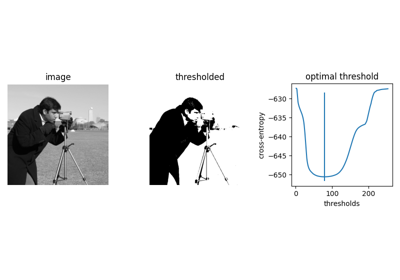## threshold_local¶

skimage.filters.threshold_local(image, block_size=3, method='gaussian', offset=0, mode='reflect', param=None, cval=0)[source]

Compute a threshold mask image based on local pixel neighborhood.

Also known as adaptive or dynamic thresholding. The threshold value is the weighted mean for the local neighborhood of a pixel subtracted by a constant. Alternatively the threshold can be determined dynamically by a given function, using the ‘generic’ method.

Parameters
image(N, M[, …, P]) ndarray

Grayscale input image.

block_sizeint or sequence of int

Odd size of pixel neighborhood which is used to calculate the threshold value (e.g. 3, 5, 7, …, 21, …).

method{‘generic’, ‘gaussian’, ‘mean’, ‘median’}, optional

Method used to determine adaptive threshold for local neighbourhood in weighted mean image.

• ‘generic’: use custom function (see param parameter)

• ‘gaussian’: apply gaussian filter (see param parameter for custom sigma value)

• ‘mean’: apply arithmetic mean filter

• ‘median’: apply median rank filter

By default the ‘gaussian’ method is used.

offsetfloat, optional

Constant subtracted from weighted mean of neighborhood to calculate the local threshold value. Default offset is 0.

mode{‘reflect’, ‘constant’, ‘nearest’, ‘mirror’, ‘wrap’}, optional

The mode parameter determines how the array borders are handled, where cval is the value when mode is equal to ‘constant’. Default is ‘reflect’.

param{int, function}, optional

Either specify sigma for ‘gaussian’ method or function object for ‘generic’ method. This functions takes the flat array of local neighbourhood as a single argument and returns the calculated threshold for the centre pixel.

cvalfloat, optional

Value to fill past edges of input if mode is ‘constant’.

Returns
threshold(N, M[, …, P]) ndarray

Threshold image. All pixels in the input image higher than the corresponding pixel in the threshold image are considered foreground.

References

1

Gonzalez, R. C. and Wood, R. E. “Digital Image Processing (2nd Edition).” Prentice-Hall Inc., 2002: 600–612. ISBN: 0-201-18075-8

Examples

>>> from skimage.data import camera
>>> image = camera()[:50, :50]
>>> binary_image1 = image > threshold_local(image, 15, 'mean')
>>> func = lambda arr: arr.mean()
>>> binary_image2 = image > threshold_local(image, 15, 'generic',
...                                         param=func)


### Examples using skimage.filters.threshold_local¶## threshold_mean¶

skimage.filters.threshold_mean(image)[source]

Return threshold value based on the mean of grayscale values.

Parameters
image(N, M[, …, P]) ndarray

Grayscale input image.

Returns
thresholdfloat

Upper threshold value. All pixels with an intensity higher than this value are assumed to be foreground.

References

1

C. A. Glasbey, “An analysis of histogram-based thresholding algorithms,” CVGIP: Graphical Models and Image Processing, vol. 55, pp. 532-537, 1993. DOI:10.1006/cgip.1993.1040

Examples

>>> from skimage.data import camera
>>> image = camera()
>>> thresh = threshold_mean(image)
>>> binary = image > thresh


### Examples using skimage.filters.threshold_mean¶## threshold_minimum¶

skimage.filters.threshold_minimum(image=None, nbins=256, max_num_iter=10000, *, hist=None)[source]

Return threshold value based on minimum method.

The histogram of the input image is computed if not provided and smoothed until there are only two maxima. Then the minimum in between is the threshold value.

Either image or hist must be provided. In case hist is given, the actual histogram of the image is ignored.

Parameters
image(N, M[, …, P]) ndarray, optional

Grayscale input image.

nbinsint, optional

Number of bins used to calculate histogram. This value is ignored for integer arrays.

max_num_iterint, optional

Maximum number of iterations to smooth the histogram.

histarray, or 2-tuple of arrays, optional

Histogram to determine the threshold from and a corresponding array of bin center intensities. Alternatively, only the histogram can be passed.

Returns
thresholdfloat

Upper threshold value. All pixels with an intensity higher than this value are assumed to be foreground.

Other Parameters
max_iterDEPRECATED

Deprecated in favor of max_num_iter.

Deprecated since version 0.19.

Raises
RuntimeError

If unable to find two local maxima in the histogram or if the smoothing takes more than 1e4 iterations.

References

1

C. A. Glasbey, “An analysis of histogram-based thresholding algorithms,” CVGIP: Graphical Models and Image Processing, vol. 55, pp. 532-537, 1993.

2

Prewitt, JMS & Mendelsohn, ML (1966), “The analysis of cell images”, Annals of the New York Academy of Sciences 128: 1035-1053 DOI:10.1111/j.1749-6632.1965.tb11715.x

Examples

>>> from skimage.data import camera
>>> image = camera()
>>> thresh = threshold_minimum(image)
>>> binary = image > thresh


### Examples using skimage.filters.threshold_minimum¶## threshold_multiotsu¶

skimage.filters.threshold_multiotsu(image=None, classes=3, nbins=256, *, hist=None)[source]

Generate classes-1 threshold values to divide gray levels in image, following Otsu’s method for multiple classes.

The threshold values are chosen to maximize the total sum of pairwise variances between the thresholded graylevel classes. See Notes and  for more details.

Either image or hist must be provided. If hist is provided, the actual histogram of the image is ignored.

Parameters
image(N, M[, …, P]) ndarray, optional

Grayscale input image.

classesint, optional

Number of classes to be thresholded, i.e. the number of resulting regions.

nbinsint, optional

Number of bins used to calculate the histogram. This value is ignored for integer arrays.

histarray, or 2-tuple of arrays, optional

Histogram from which to determine the threshold, and optionally a corresponding array of bin center intensities. If no hist provided, this function will compute it from the image (see notes).

Returns
thresharray

Array containing the threshold values for the desired classes.

Raises
ValueError

If image contains less grayscale value then the desired number of classes.

Notes

This implementation relies on a Cython function whose complexity is $$O\left(\frac{Ch^{C-1}}{(C-1)!}\right)$$, where $$h$$ is the number of histogram bins and $$C$$ is the number of classes desired.

If no hist is given, this function will make use of skimage.exposure.histogram, which behaves differently than np.histogram. While both allowed, use the former for consistent behaviour.

The input image must be grayscale.

References

1

Liao, P-S., Chen, T-S. and Chung, P-C., “A fast algorithm for multilevel thresholding”, Journal of Information Science and Engineering 17 (5): 713-727, 2001. Available at: <https://ftp.iis.sinica.edu.tw/JISE/2001/200109_01.pdf> DOI:10.6688/JISE.2001.17.5.1

2

Tosa, Y., “Multi-Otsu Threshold”, a java plugin for ImageJ. Available at: <http://imagej.net/plugins/download/Multi_OtsuThreshold.java>

Examples

>>> from skimage.color import label2rgb
>>> from skimage import data
>>> image = data.camera()
>>> thresholds = threshold_multiotsu(image)
>>> regions = np.digitize(image, bins=thresholds)
>>> regions_colorized = label2rgb(regions)


### Examples using skimage.filters.threshold_multiotsu¶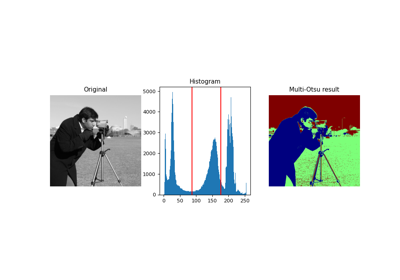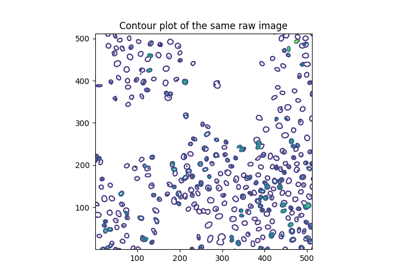## threshold_niblack¶

skimage.filters.threshold_niblack(image, window_size=15, k=0.2)[source]

Applies Niblack local threshold to an array.

A threshold T is calculated for every pixel in the image using the following formula:

T = m(x,y) - k * s(x,y)


where m(x,y) and s(x,y) are the mean and standard deviation of pixel (x,y) neighborhood defined by a rectangular window with size w times w centered around the pixel. k is a configurable parameter that weights the effect of standard deviation.

Parameters
image(N, M[, …, P]) ndarray

Grayscale input image.

window_sizeint, or iterable of int, optional

Window size specified as a single odd integer (3, 5, 7, …), or an iterable of length image.ndim containing only odd integers (e.g. (1, 5, 5)).

kfloat, optional

Value of parameter k in threshold formula.

Returns
threshold(N, M) ndarray

Threshold mask. All pixels with an intensity higher than this value are assumed to be foreground.

Notes

This algorithm is originally designed for text recognition.

The Bradley threshold is a particular case of the Niblack one, being equivalent to

>>> from skimage import data
>>> image = data.page()
>>> q = 1
>>> threshold_image = threshold_niblack(image, k=0) * q


for some value q. By default, Bradley and Roth use q=1.

References

1

W. Niblack, An introduction to Digital Image Processing, Prentice-Hall, 1986.

2

D. Bradley and G. Roth, “Adaptive thresholding using Integral Image”, Journal of Graphics Tools 12(2), pp. 13-21, 2007. DOI:10.1080/2151237X.2007.10129236

Examples

>>> from skimage import data
>>> image = data.page()
>>> threshold_image = threshold_niblack(image, window_size=7, k=0.1)


### Examples using skimage.filters.threshold_niblack¶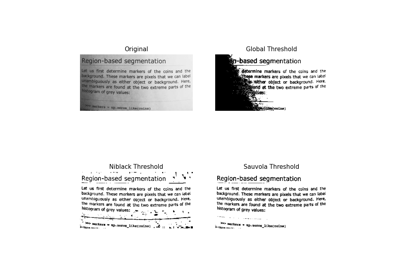## threshold_otsu¶

skimage.filters.threshold_otsu(image=None, nbins=256, *, hist=None)[source]

Return threshold value based on Otsu’s method.

Either image or hist must be provided. If hist is provided, the actual histogram of the image is ignored.

Parameters
image(N, M[, …, P]) ndarray, optional

Grayscale input image.

nbinsint, optional

Number of bins used to calculate histogram. This value is ignored for integer arrays.

histarray, or 2-tuple of arrays, optional

Histogram from which to determine the threshold, and optionally a corresponding array of bin center intensities. If no hist provided, this function will compute it from the image.

Returns
thresholdfloat

Upper threshold value. All pixels with an intensity higher than this value are assumed to be foreground.

Notes

The input image must be grayscale.

References

1

Examples

>>> from skimage.data import camera
>>> image = camera()
>>> thresh = threshold_otsu(image)
>>> binary = image <= thresh


### Examples using skimage.filters.threshold_otsu¶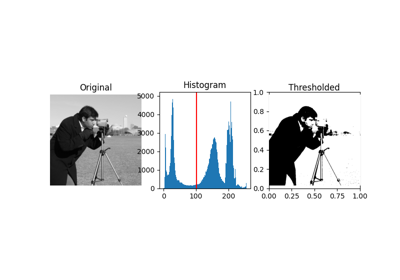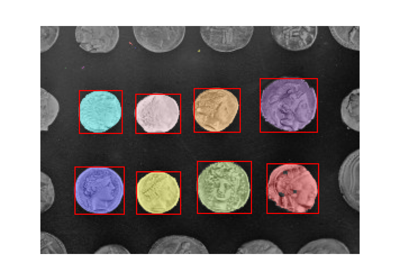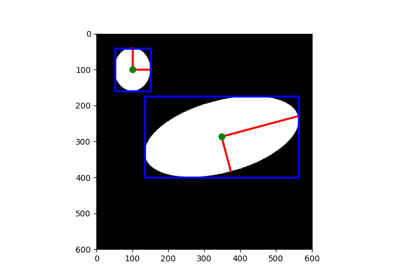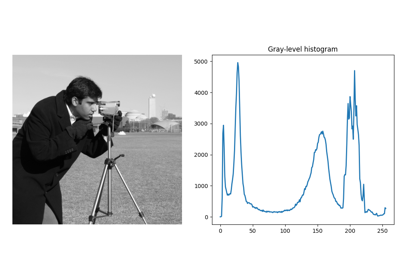## threshold_sauvola¶

skimage.filters.threshold_sauvola(image, window_size=15, k=0.2, r=None)[source]

Applies Sauvola local threshold to an array. Sauvola is a modification of Niblack technique.

In the original method a threshold T is calculated for every pixel in the image using the following formula:

T = m(x,y) * (1 + k * ((s(x,y) / R) - 1))


where m(x,y) and s(x,y) are the mean and standard deviation of pixel (x,y) neighborhood defined by a rectangular window with size w times w centered around the pixel. k is a configurable parameter that weights the effect of standard deviation. R is the maximum standard deviation of a grayscale image.

Parameters
image(N, M[, …, P]) ndarray

Grayscale input image.

window_sizeint, or iterable of int, optional

Window size specified as a single odd integer (3, 5, 7, …), or an iterable of length image.ndim containing only odd integers (e.g. (1, 5, 5)).

kfloat, optional

Value of the positive parameter k.

rfloat, optional

Value of R, the dynamic range of standard deviation. If None, set to the half of the image dtype range.

Returns
threshold(N, M) ndarray

Threshold mask. All pixels with an intensity higher than this value are assumed to be foreground.

Notes

This algorithm is originally designed for text recognition.

References

1

J. Sauvola and M. Pietikainen, “Adaptive document image binarization,” Pattern Recognition 33(2), pp. 225-236, 2000. DOI:10.1016/S0031-3203(99)00055-2

Examples

>>> from skimage import data
>>> image = data.page()
>>> t_sauvola = threshold_sauvola(image, window_size=15, k=0.2)
>>> binary_image = image > t_sauvola


### Examples using skimage.filters.threshold_sauvola¶## threshold_triangle¶

skimage.filters.threshold_triangle(image, nbins=256)[source]

Return threshold value based on the triangle algorithm.

Parameters
image(N, M[, …, P]) ndarray

Grayscale input image.

nbinsint, optional

Number of bins used to calculate histogram. This value is ignored for integer arrays.

Returns
thresholdfloat

Upper threshold value. All pixels with an intensity higher than this value are assumed to be foreground.

References

1

Zack, G. W., Rogers, W. E. and Latt, S. A., 1977, Automatic Measurement of Sister Chromatid Exchange Frequency, Journal of Histochemistry and Cytochemistry 25 (7), pp. 741-753 DOI:10.1177/25.7.70454

2

ImageJ AutoThresholder code, http://fiji.sc/wiki/index.php/Auto_Threshold

Examples

>>> from skimage.data import camera
>>> image = camera()
>>> thresh = threshold_triangle(image)
>>> binary = image > thresh


## threshold_yen¶

skimage.filters.threshold_yen(image=None, nbins=256, *, hist=None)[source]

Return threshold value based on Yen’s method. Either image or hist must be provided. In case hist is given, the actual histogram of the image is ignored.

Parameters
image(N, M[, …, P]) ndarray

Grayscale input image.

nbinsint, optional

Number of bins used to calculate histogram. This value is ignored for integer arrays.

histarray, or 2-tuple of arrays, optional

Histogram from which to determine the threshold, and optionally a corresponding array of bin center intensities. An alternative use of this function is to pass it only hist.

Returns
thresholdfloat

Upper threshold value. All pixels with an intensity higher than this value are assumed to be foreground.

References

1

Yen J.C., Chang F.J., and Chang S. (1995) “A New Criterion for Automatic Multilevel Thresholding” IEEE Trans. on Image Processing, 4(3): 370-378. DOI:10.1109/83.366472

2

Sezgin M. and Sankur B. (2004) “Survey over Image Thresholding Techniques and Quantitative Performance Evaluation” Journal of Electronic Imaging, 13(1): 146-165, DOI:10.1117/1.1631315 http://www.busim.ee.boun.edu.tr/~sankur/SankurFolder/Threshold_survey.pdf

3

ImageJ AutoThresholder code, http://fiji.sc/wiki/index.php/Auto_Threshold

Examples

>>> from skimage.data import camera
>>> image = camera()
>>> thresh = threshold_yen(image)
>>> binary = image <= thresh


## try_all_threshold¶

skimage.filters.try_all_threshold(image, figsize=(8, 5), verbose=True)[source]

Returns a figure comparing the outputs of different thresholding methods.

Parameters
image(N, M) ndarray

Input image.

figsizetuple, optional

Figure size (in inches).

verbosebool, optional

Print function name for each method.

Returns
fig, axtuple

Matplotlib figure and axes.

Notes

The following algorithms are used:

• isodata

• li

• mean

• minimum

• otsu

• triangle

• yen

Examples

>>> from skimage.data import text
>>> fig, ax = try_all_threshold(text(), figsize=(10, 6), verbose=False)


### Examples using skimage.filters.try_all_threshold¶skimage.filters.unsharp_mask(image, radius=1.0, amount=1.0, multichannel=False, preserve_range=False, *, channel_axis=None)[source]

The sharp details are identified as the difference between the original image and its blurred version. These details are then scaled, and added back to the original image.

Parameters
image[P, …, ]M[, N][, C] ndarray

Input image.

radiusscalar or sequence of scalars, optional

If a scalar is given, then its value is used for all dimensions. If sequence is given, then there must be exactly one radius for each dimension except the last dimension for multichannel images. Note that 0 radius means no blurring, and negative values are not allowed.

amountscalar, optional

The details will be amplified with this factor. The factor could be 0 or negative. Typically, it is a small positive number, e.g. 1.0.

multichannelbool, optional

If True, the last image dimension is considered as a color channel, otherwise as spatial. Color channels are processed individually. This argument is deprecated: specify channel_axis instead.

preserve_rangebool, optional

Whether to keep the original range of values. Otherwise, the input image is converted according to the conventions of img_as_float. Also see https://scikit-image.org/docs/dev/user_guide/data_types.html

channel_axisint or None, optional

If None, the image is assumed to be a grayscale (single channel) image. Otherwise, this parameter indicates which axis of the array corresponds to channels.

New in version 0.19: channel_axis was added in 0.19.

Returns
output[P, …, ]M[, N][, C] ndarray of float

Other Parameters
multichannelDEPRECATED

Deprecated in favor of channel_axis.

Deprecated since version 0.19.

Notes

Unsharp masking is an image sharpening technique. It is a linear image operation, and numerically stable, unlike deconvolution which is an ill-posed problem. Because of this stability, it is often preferred over deconvolution.

The main idea is as follows: sharp details are identified as the difference between the original image and its blurred version. These details are added back to the original image after a scaling step:

enhanced image = original + amount * (original - blurred)

When applying this filter to several color layers independently, color bleeding may occur. More visually pleasing result can be achieved by processing only the brightness/lightness/intensity channel in a suitable color space such as HSV, HSL, YUV, or YCbCr.

Unsharp masking is described in most introductory digital image processing books. This implementation is based on .

References

1

Maria Petrou, Costas Petrou “Image Processing: The Fundamentals”, (2010), ed ii., page 357, ISBN 13: 9781119994398 DOI:10.1002/9781119994398

2

Examples

>>> array = np.ones(shape=(5,5), dtype=np.uint8)*100
>>> array[2,2] = 120
>>> array
array([[100, 100, 100, 100, 100],
[100, 100, 100, 100, 100],
[100, 100, 120, 100, 100],
[100, 100, 100, 100, 100],
[100, 100, 100, 100, 100]], dtype=uint8)
array([[0.39, 0.39, 0.39, 0.39, 0.39],
[0.39, 0.39, 0.38, 0.39, 0.39],
[0.39, 0.38, 0.53, 0.38, 0.39],
[0.39, 0.39, 0.38, 0.39, 0.39],
[0.39, 0.39, 0.39, 0.39, 0.39]])

>>> array = np.ones(shape=(5,5), dtype=np.int8)*100
>>> array[2,2] = 127
array([[0.79, 0.79, 0.79, 0.79, 0.79],
[0.79, 0.78, 0.75, 0.78, 0.79],
[0.79, 0.75, 1.  , 0.75, 0.79],
[0.79, 0.78, 0.75, 0.78, 0.79],
[0.79, 0.79, 0.79, 0.79, 0.79]])

>>> np.around(unsharp_mask(array, radius=0.5, amount=2, preserve_range=True), 2)
array([[100.  , 100.  ,  99.99, 100.  , 100.  ],
[100.  ,  99.39,  95.48,  99.39, 100.  ],
[ 99.99,  95.48, 147.59,  95.48,  99.99],
[100.  ,  99.39,  95.48,  99.39, 100.  ],
[100.  , 100.  ,  99.99, 100.  , 100.  ]])


### Examples using skimage.filters.unsharp_mask¶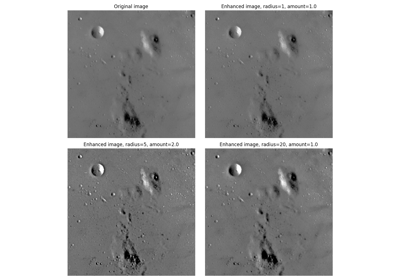## wiener¶

skimage.filters.wiener(data, impulse_response=None, filter_params={}, K=0.25, predefined_filter=None)[source]

Minimum Mean Square Error (Wiener) inverse filter.

Parameters
data(M,N) ndarray

Input data.

Kfloat or (M,N) ndarray

Ratio between power spectrum of noise and undegraded image.

impulse_responsecallable f(r, c, **filter_params)

Impulse response of the filter. See LPIFilter2D.__init__.

filter_paramsdict

Additional keyword parameters to the impulse_response function.

Other Parameters
predefined_filterLPIFilter2D

If you need to apply the same filter multiple times over different images, construct the LPIFilter2D and specify it here.

## window¶

skimage.filters.window(window_type, shape, warp_kwargs=None)[source]

Return an n-dimensional window of a given size and dimensionality.

Parameters
window_typestring, float, or tuple

The type of window to be created. Any window type supported by scipy.signal.get_window is allowed here. See notes below for a current list, or the SciPy documentation for the version of SciPy on your machine.

shapetuple of int or int

The shape of the window along each axis. If an integer is provided, a 1D window is generated.

warp_kwargsdict

Keyword arguments passed to skimage.transform.warp (e.g., warp_kwargs={'order':3} to change interpolation method).

Returns
nd_windowndarray

A window of the specified shape. dtype is np.double.

Notes

This function is based on scipy.signal.get_window and thus can access all of the window types available to that function (e.g., "hann", "boxcar"). Note that certain window types require parameters that have to be supplied with the window name as a tuple (e.g., ("tukey", 0.8)). If only a float is supplied, it is interpreted as the beta parameter of the Kaiser window.

Note that this function generates a double precision array of the specified shape and can thus generate very large arrays that consume a large amount of available memory.

The approach taken here to create nD windows is to first calculate the Euclidean distance from the center of the intended nD window to each position in the array. That distance is used to sample, with interpolation, from a 1D window returned from scipy.signal.get_window. The method of interpolation can be changed with the order keyword argument passed to skimage.transform.warp.

Some coordinates in the output window will be outside of the original signal; these will be filled in with zeros.

Window types: - boxcar - triang - blackman - hamming - hann - bartlett - flattop - parzen - bohman - blackmanharris - nuttall - barthann - kaiser (needs beta) - gaussian (needs standard deviation) - general_gaussian (needs power, width) - slepian (needs width) - dpss (needs normalized half-bandwidth) - chebwin (needs attenuation) - exponential (needs decay scale) - tukey (needs taper fraction)

References

1

Two-dimensional window design, Wikipedia, https://en.wikipedia.org/wiki/Two_dimensional_window_design

Examples

Return a Hann window with shape (512, 512):

>>> from skimage.filters import window
>>> w = window('hann', (512, 512))


Return a Kaiser window with beta parameter of 16 and shape (256, 256, 35):

>>> w = window(16, (256, 256, 35))


Return a Tukey window with an alpha parameter of 0.8 and shape (100, 300):

>>> w = window(('tukey', 0.8), (100, 300))


### Examples using skimage.filters.window¶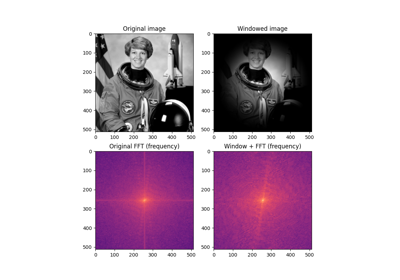## LPIFilter2D¶

class skimage.filters.LPIFilter2D(impulse_response, **filter_params)[source]

Bases: object

Linear Position-Invariant Filter (2-dimensional)

__init__(impulse_response, **filter_params)[source]
Parameters
impulse_responsecallable f(r, c, **filter_params)

Function that yields the impulse response. r and c are 1-dimensional vectors that represent row and column positions, in other words coordinates are (r,c),(r,c) etc. **filter_params are passed through.

In other words, impulse_response would be called like this:

>>> def impulse_response(r, c, **filter_params):
...     pass
>>>
>>> r = [0,0,0,1,1,1,2,2,2]
>>> c = [0,1,2,0,1,2,0,1,2]
>>> filter_params = {'kw1': 1, 'kw2': 2, 'kw3': 3}
>>> impulse_response(r, c, **filter_params)


Examples

Gaussian filter: Use a 1-D gaussian in each direction without normalization coefficients.

>>> def filt_func(r, c, sigma = 1):
...     return np.exp(-np.hypot(r, c)/sigma)
>>> filter = LPIFilter2D(filt_func)